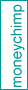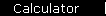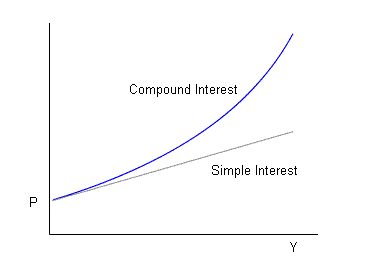# Simple Interest Calculator

Compound Interest means that you earn "interest on your interest", while Simple Interest means that you don't - your interest payments stay constant, at a fixed percentage of the original principal. First, a calculator to let you see the difference.

 Inputs
Starting Principal: \$
Years:
Annual Interest Rate:   %

 Results
Future Value, using...
Simple Interest: \$
Annually Compounded Interest: \$

The lesson is that compound interest is a better investment, which seems both obvious and moot - after all, bank accounts always pay compound interest anyway. Even a bond investment is really compound interest if you think about it: you get fixed coupons (that's simple interest) but you can invest them to get interest on them (ergo compound interest).

The situation where simple interest occurs naturally is when the principal doesn't change over time. This is true with an interest-only mortgage, for example, where your monthly payments only pay the interest on your loan, but don't pay down the loan itself.

## Simple Interest Formula

Lets say that P is your starting principal (spelled -pal and not -ple, because Your Money is Your Pal), r is the interest rate (expressed as a decimal), and Y is the number of years you invest. Then your future value will be:

 P (1 + rY) (Simple Interest) P (1 + r)Y (Annually Compounded Interest)

Note the two formulas give the same answer for one year. After that, compound interest takes off.home  |  formulas  |  glossary  |  calculator  |  about us  |  books

copyright © www.moneychimp.com   . . . . . . . . . .   licensingAlso See...